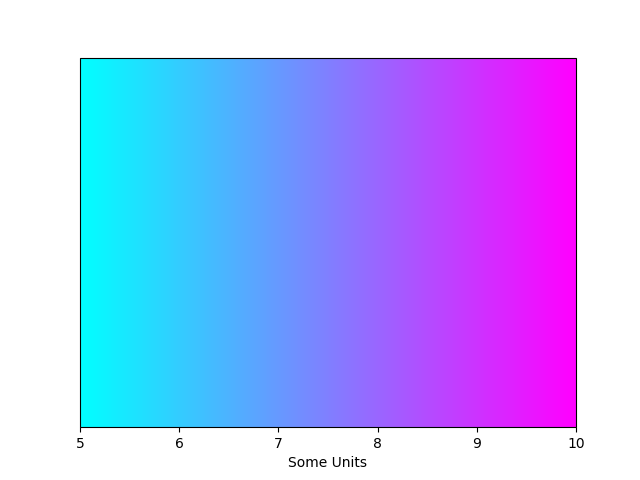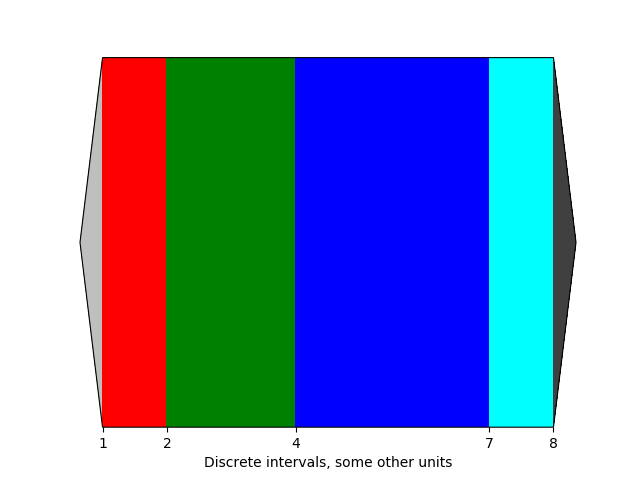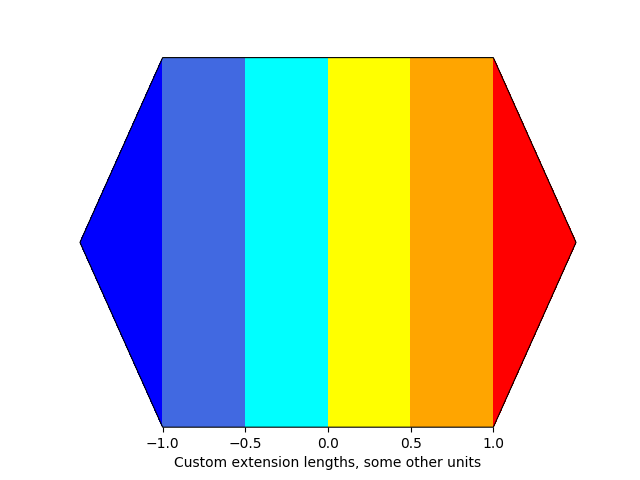Travis-CI:# Customized Colorbars Tutorial¶

This tutorial shows how to build colorbars without an attached plot.

## Customized Colorbars¶

`ColorbarBase` derives from `ScalarMappable` and puts a colorbar in a specified axes, so it has everything needed for a standalone colorbar. It can be used as is to make a colorbar for a given colormap and does not need a mappable object like an image. In this tutorial we will explore what can be done with standalone colorbar.

### Basic continuous colorbar¶

Set the colormap and norm to correspond to the data for which the colorbar will be used. Then create the colorbar by calling `ColorbarBase` and specify axis, colormap, norm and orientation as parameters. Here we create a basic continuous colorbar with ticks and labels. More information on colorbar api can be found `here`.

```import matplotlib.pyplot as plt
import matplotlib as mpl

fig, ax = plt.subplots()

cmap = mpl.cm.cool
norm = mpl.colors.Normalize(vmin=5, vmax=10)

cb1 = mpl.colorbar.ColorbarBase(ax, cmap=cmap,
norm=norm,
orientation='horizontal')
cb1.set_label('Some Units')
fig.show()
```### Discrete intervals colorbar¶

The second example illustrates the use of a `ListedColormap` which generates a colormap from a set of listed colors, `colors.BoundaryNorm()` which generates a colormap index based on discrete intervals and extended ends to show the “over” and “under” value colors. Over and under are used to display data outside of the normalized [0,1] range. Here we pass colors as gray shades as a string encoding a float in the 0-1 range.

If a `ListedColormap` is used, the length of the bounds array must be one greater than the length of the color list. The bounds must be monotonically increasing.

This time we pass some more arguments in addition to previous arguments to `ColorbarBase`. For the out-of-range values to display on the colorbar, we have to use the extend keyword argument. To use extend, you must specify two extra boundaries. Finally spacing argument ensures that intervals are shown on colorbar proportionally.

```fig, ax = plt.subplots()

cmap = mpl.colors.ListedColormap(['red', 'green', 'blue', 'cyan'])
cmap.set_over('0.25')
cmap.set_under('0.75')

bounds = [1, 2, 4, 7, 8]
norm = mpl.colors.BoundaryNorm(bounds, cmap.N)
cb2 = mpl.colorbar.ColorbarBase(ax, cmap=cmap,
norm=norm,
boundaries= + bounds + ,
extend='both',
ticks=bounds,
spacing='proportional',
orientation='horizontal')
cb2.set_label('Discrete intervals, some other units')
fig.show()
```### Colorbar with custom extension lengths¶

Here we illustrate the use of custom length colorbar extensions, used on a colorbar with discrete intervals. To make the length of each extension same as the length of the interior colors, use `extendfrac='auto'`.

```fig, ax = plt.subplots()

cmap = mpl.colors.ListedColormap(['royalblue', 'cyan',
'yellow', 'orange'])
cmap.set_over('red')
cmap.set_under('blue')

bounds = [-1.0, -0.5, 0.0, 0.5, 1.0]
norm = mpl.colors.BoundaryNorm(bounds, cmap.N)
cb3 = mpl.colorbar.ColorbarBase(ax, cmap=cmap,
norm=norm,
boundaries=[-10] + bounds + ,
extend='both',
extendfrac='auto',
ticks=bounds,
spacing='uniform',
orientation='horizontal')
cb3.set_label('Custom extension lengths, some other units')
fig.show()
```Total running time of the script: ( 0 minutes 0.064 seconds)

Gallery generated by Sphinx-Gallery